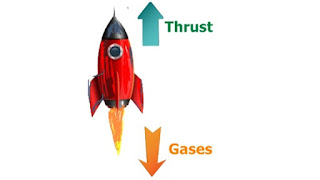Instructed by: Vishesh Nigam | Subject: Academics , Math & ScienceThis chapter introduces you to more advanced concepts like collision in 2 dimension or how momentum is conserved when mass of a body reduces during the motion. The much confused "Rocket equations" is tackled in a way that makes understanding the concept really easy. The famous bullet - pendulum problem is also explained in a way that the concept of energy and momentum conservation is combined in a very useful way

### InstructorVishesh Nigam

My love for physics and teaching has been lifelong. Here are my 8 suggestions on how you can get good at the subject- 1. You will need to make consistent effort. Start by learning the theory well before you do numerical 2. Visualize Physics- Making mental pictures of concepts as you study them and imagining the possibilities can really speed up understanding of it. E.g. if you are reading static and dynamic friction, imagine what is happening to a block placed on an inclined plane as the incline is increasing. 3. Try to absorb the contents of a tutorial in small chunks. Pause and ponder over what you read. Visualize and scribble on a paper 4. Understand the derivation of important equations - Often the derivation itself gives great clarity around the topic and the numerical problems you will attempt. 5. Learn to deconstruct a numerical problem. Making neat diagrams and putting all the available information around it can accelerate you towards writing the correct equations and therefore the answers 6. Be disciplined about not "rushing through" when solving a numerical problem. In other words, do not jump steps while doing a problem. 7. Get the basic understanding of math, particular integral and differential calculus, in place. It will be required for solving numerical problems 8. Revisit the chapter after you have completed numerical. You'll find your understanding of the topic improve several notches

### Course Info

Center of Mass and Linear Momentum (Part 2)Reviewed by Acamig Courses on November 19, 2018 Rating: 5